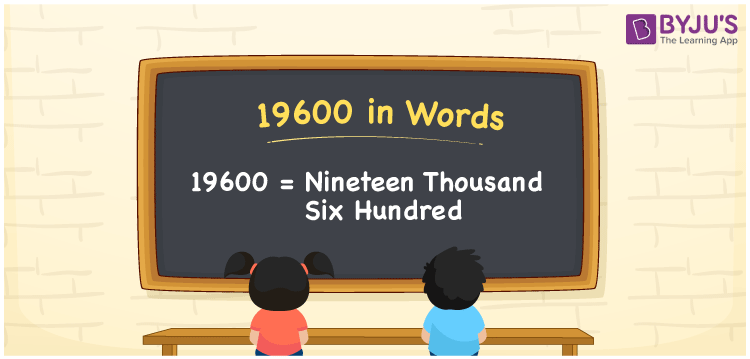# 19600 in Words

We can write 19600 in words as Nineteen thousand six hundred. Writing a number in word form is an easy task if we use a place value concept. We can quickly write any number in words using the place value chart. Also, the word form of numbers means the language we use to spell that particular number. Suppose to convey the cardinal number 19600 through any medium, and we spell it as Nineteen thousand six hundred.

19600 in words

Nineteen thousand six hundred

Nineteen thousand six hundred in Numbers

19600

## 19600 in English Words

Generally, we use the English alphabet to write numbers in words. Thus, we can write 19600 in English words as “Nineteen thousand six hundred”.## How to Write 19600 in Words?

As mentioned above, the conversion of a number to word form is simple with the help of a place value chart. Let’s understand how to write 19600 in words using the table below.

 Ten thousands Thousands Hundreds Tens Ones 1 9 6 0 0

Here, ones = 0, tens = 0, hundreds = 6, thousands = 9, ten thousands = 1

So, these numbers can be expanded as:

1 × Ten thousand + 9 × Thousand + 6 × Hundred + 0 × Ten + 0 × One

= 1 × 10000 + 9 × 1000 + 6 × 100 + 0 × 10 + 0 × 1

= 10000 + 9000 + 600

= Ten Thousand + Nine thousand + Six hundred

= Nineteen thousand + Six hundred {since ten + nine = nineteen}

= Nineteen thousand six hundred

As we know, 19600 is a natural number. Also, it is the successor of 19599 and predecessor of 19601.

19600 in words – Nineteen thousand six hundred

Is 19600 an odd number? – No

Is 19600 an even number? – Yes

Is 19600 a prime number? – No

Is 19600 a composite number? – Yes

Is 19600 a perfect square number? – Yes

What is the square root of 19600? – 140

Is 19600 a perfect cube number? – No

## Frequently Asked Questions on 19600 in Words

Q1

### How do you write 19600 in words?

We can write the number 19600 in words as Nineteen thousand six hundred.
Q2

### How to write Rs. 19600 on a cheque?

On a cheque, Rs. 19600 can be written as “Nineteen thousand six hundred rupees only”.
Q3

### Find the value of 14000 + 5600. Write the answer in words.

14000 + 5600 = 19600 The value of 14000 + 5600 is 19600, and it is written in words as Nineteen thousand six hundred.